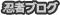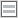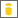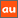0除算に関するアナウンスメントを公開しています

The purpose of this site is to publish announcements on the theory of division by zero

Announcement 326: The division by zero $z/0=0$ - its impact to human beings through education and research

Institute of Reproducing Kernels
Kawauchi-cho, 5-1648-16,
Kiryu 376-0041, Japan
E-mail: kbdmm360@yahoo.co.jp
October 17, 2016

Abstract: In this announcement, for its importance we would like to state the situation on the division by zero and propose basic new challenges to education and research on our wrong world history.

1 Introduction
By a natural extension of the fractions
\begin{equation}
\frac{b}{a} \tag{1.1}
\end{equation}for any complex numbers $a$ and $b$, we found the simple and beautiful result, for any complex number $b$
\begin{equation}
\frac{b}{0}=0, \tag{1.2}
\end{equation}incidentally in  by the Tikhonov regularization for the Hadamard product inversions for matrices, and we discussed their properties and gave several physical interpretations on the general fractions in  for the case of real numbers.
The division by zero has a long and mysterious story over the world $($see, for example, Google site with division by zero$)$ with its physical viewpoints since the document of zero in India on AD 628, however, Sin-Ei, Takahasi   established a simple and decisive interpretation $($1.2$)$ by analyzing the extensions of fractions and by showing the complete characterization for the property $($1.2$)$:

Proposition. Let F be a function from ${\bf C }\times {\bf C }$ to ${\bf C }$ satisfying
\begin{equation}
F (b, a)F (c, d)= F (bc, ad)
\end{equation}for all $a, b, c, d \in {\bf C }$, and
$$F (b, a) = \frac {b}{a }, \quad a, b \in {\bf C }, a \ne 0.$$Then, we obtain, for any $b \in {\bf C }$
$F (b, 0) = 0.$
Note that the complete proof of this proposition is simply given by  2 or 3 lines.  We should define $F(b,0)=b/0=0$ in general.
We thus should consider, for any complex number $b$, as $($1.2$)$; that is , for the mapping
\begin{equation}
w = \frac{1}{z}, \tag{1.3}
\end{equation}the image of $z=0$ is $w=0$ $($should be defined$)$. This fact seems to be a curious one in connection with our well-established popular image for the  point at infinity on the Riemann sphere. Therefore, the division by zero will give great impacts to complex analysis and to our ideas for the space and universe.
However, the division by zero $($1.2$)$ is now clear, indeed, for the introduction of $($1.2$)$, we have several independent approaches as in:
1) by the generalization of the fractions by the Tikhonov regularization and by the Moore-Penrose generalized inverse,
2) by the intuitive meaning of the fractions $($division$)$ by H. Michiwaki - repeated subtraction method,
3) by the unique extension of the fractions by S. Takahasi,   as in the above,
4) by the extension of the fundamental function $W = 1/z$ from ${\bf C} \setminus \{0\}$ into ${\bf C}$ such that $W =1/z$ is a one to one and onto mapping from ${\bf C} \setminus \{0\}$ onto ${\bf C} \setminus \{0\}$ and the division by zero $1/0=0$ is a one to one and onto mapping extension of the function $W =1/z$ from  ${\bf C}$ onto ${\bf C}$,
and
5) by considering the values of functions with the mean values of functions.
Furthermore, in  we gave the results in order to show the reality of the division by zero in our world:
A) a field structure  containing the division by zero --- the Yamada field ${\bf Y}$,
B)  by the gradient of the $y$ axis on the $(x,y)$ plane --- $\tan \frac{\pi}{2} =0$,
C) by the reflection $W =1/\overline{z}$ of $W= z$ with respect to the unit circle with center at the origin on the complex $z$ plane --- the reflection point of zero is zero, not the point at infinity.
And
D) by considering rotation of a right circular cone having some very interesting phenomenon  from some practical and physical problem.
In ,  many division by zero results in Euclidean spaces are given and  the basic idea at the point at infinity should be changed. In , we gave beautiful geometrical interpretations of determinants from the viewpoint of the division by zero. The results show that the division by zero is our basic and elementary mathematics in our world.
See  J. A. Bergstra, Y. Hirshfeld and J. V. Tucker  for the relationship between fields and the division by zero, and the importance of the division by zero for computer science. It seems that the relationship of the division by zero and field structures are abstract in their paper.
Meanwhile,  J. P.  Barukcic and I.  Barukcic  discussed recently the relation between the divisions $0/0$, $1/0$ and special relative theory of Einstein. However, their logic seems to be curious and their results contradict with ours.
Furthermore,  T. S. Reis and J.A.D.W. Anderson [8,9] extend the system of the real numbers by introducing an ideal number for the division by zero $0/0$.
Meanwhile, we should refer to up-to-date information:
Riemann Hypothesis Addendum - Breakthrough
Kurt Arbenz
https://www.researchgate.net/publication/272022137 Riemann Hypothesis Addendum -   Breakthrough.
Here, we recall Albert Einstein's words on mathematics: Blackholes are where God divided by zero. I don't believe in mathematics. George Gamow $($1904-1968$)$ Russian-born American nuclear physicist and cosmologist remarked that "it is well known to students of high school algebra" that division by zero is not valid; and Einstein admitted it as the biggest blunder of his life  1. Gamow, G., My World Line $($Viking, New York$)$. p 44, 1970.
Apparently, the division by zero is a great missing in our mathematics and the result $(1.2)$ is definitely determined as our basic mathematics, as we see from Proposition 1.  Note  its very general assumptions and  many fundamental evidences in our world in [4,6,7]. The results will give great impact  on Euclidean spaces, analytic geometry, calculus, differential equations, complex analysis and  physical problems.
The mysterious history of the division by zero over one thousand years is a great shame of  mathematicians and human race on the world history, like the Ptolemaic system $($geocentric theory$)$. The division by zero will become a typical  symbol of foolish human race with long and unceasing struggles. Future people will realize this fact as a definite common sense.
We should check and fill our mathematics, globally and beautifully, from the viewpoint of the division by zero. Our mathematics will be more perfect and beautiful,  and will give great impact to our basic ideas on the universe.
For our ideas on the division by zero, see the survey style announcements.

2 Basic Materials of Mathematics

$(1)$: First, we should declare that the divison by zero is possible in the natural and uniquley determined sense and its importance.

$(2)$: In the elementary school, we should introduce the concept of division by the idea of repeated subtraction method by H. Michiwaki whoes method is applied in computer algorithmu and in old days for calculation of division. This method will give a simple and clear method for calculation of division and students will be happy to apply this simple method at the first stage. At this time, they will be able to understand that the division by zero is clear and trivial as $a/0=0$ for any $a$. Note that Michiwaki knows how to apply his method to the complex number field.

$(3)$: For the introduction of the elemetary function $y= 1/x$, we should give the definition of the function at the origin $x=0$ as $y = 0$ by the division by zero idea and we should apply this definition for the occasions of its appearences, step by step, following the curriculum and the results of the division by zero.

$(4)$: For the idea of the Euclidean space (plane), we should introduce, at the first stage, the concept of steleographic projection and the concept of the point at infinity  -  one point compactification. Then, we will be able to see the whole Euclidean plane, however, by the division by zero, the point at infinity is represented by zero. We can teach  the very important fact with many geometric and analytic geometry methods. These topics will give great pleasant feelings to many students. Interesting topics are: parallel lines, what is a line? - a line contains the origin as an isolated point for the case that the native line does not through the origin. All the lines pass the origin, our space is not the Eulcildean space and is not Aristoteles for the strong discontinuity at the point at infinity (at the origin). - Here note that an orthogonal coordinates should be fixed first for our all arguments.

$(5)$: The inversion of the origin with respect to a circle with center the origin is the origin itself, not the point at infinity - the very classical result is wrong. We can also prove this elementary result by many elementary ways.

$(6)$: We should change the concept of gradients; on the usual orthogonal coordinates $(x,y)$,  the gradient of the $y$ axis is zero; this is given and proved by the fundamental result  $\tan (\pi/2) =0$. The result is trivial in the definition of the Yamada field. This result is derived also from  the division by zero calculus:

For any formal Laurent expansion around $z=a$,
\begin{equation}
f(z) = \sum_{n=-\infty}^{\infty} C_n (z - a)^n,\tag{2,1}
\end{equation}
we obtain the identity, by the division by zero

\begin{equation}
f(a) =  C_0. \tag{2,2}
\end{equation}
This fundamental result leads to the important new definition:
From the viewpoint of the division by zero, when there exists the limit, at $x$
\begin{equation}
f^\prime(x) = \lim_{h\to 0} \frac{f(x + h) - f(x)}{h}  =\infty \tag{2,3}
\end{equation}
or
\begin{equation}
f^\prime(x) =  -\infty, \tag{2,4}
\end{equation}
both cases, we can write them as follows:
\begin{equation}
f^\prime(x) =  0. \tag{2,5}
\end{equation}

For the elementary ordinary differential equation
\begin{equation}
y^\prime = \frac{dy}{dx} =\frac{1}{x}, \quad x > 0, \tag{2,6}
\end{equation}
how will be the case at the point $x = 0$? From its general solution, with a general constant $C$
\begin{equation}
y = \log x + C, \tag{2,7}
\end{equation}
we see that, by the division by zero,
\begin{equation}
y^\prime (0)= \left[ \frac{1}{x}\right]_{x=0} = 0, \tag{2,8}
\end{equation}
that will mean that the division by zero (1.2) is very natural.
In addition, note that the function $y = \log x$ has infinite order derivatives and all the values are zero at the origin, in the sense of the division by zero.
However, for the derivative of the function $y = \log x$, we have to fix the sense at the origin, clearly, because the function is not differentiable, but it has a singularity at the origin. For $x >0$, there is no problem for (2.6) and (2.7). At  $x = 0$, we  see that we can not consider the limit in the sense (2.3).  However,  for $x >0$ we have (2.6) and
\begin{equation}
\lim_{x \to +0} \left(\log x \right)^\prime = +\infty. \tag{2,9}
\end{equation}
In the usual sense, the limit is $+\infty$,  but in the present case, in the sense of the division by zero, we have:
\begin{equation}
\left[ \left(\log x \right)^\prime \right]_{x=0}= 0 \tag{2,10}
\end{equation}
and we will be able to understand its sense graphycally.

By the new interpretation for the derivative, we can arrange many formulas for derivatives, by the division by zero. We can modify many formulas and statements in calculus and we can apply our concept to the differential equation theory and the universe in connetion with derivatives.

$(7)$: We shall introduce the typical division by zero calculus.
For the integral
\begin{equation}
\end{equation}
we obtain, by the division by zero,
\begin{equation}
\int x(x^{2}+1)^{-1}dx=\frac{\log(x^{2}+1)}{2}. \tag{2,12}
\end{equation}
We will consider the fundamental ordinary differential equations
\begin{equation}
x^{\prime \prime}(t) =g -kx^{\prime}(t) \tag{2,13}
\end{equation}
with the initial conditions
\begin{equation}
x(0)  = -h, x^{\prime}(0) =0. \tag{2,14}
\end{equation}
Then we have the solution
\begin{equation}
x(t) = \frac{g}{k}t + \frac{g(e^{-kt}- 1)}{k^2} - h. \tag{2,15}
\end{equation}
Then, for $k=0$, we obtain, immediately, by the division by zero
\begin{equation}
x(t) = \frac{1}{2}g t^2 -h. \tag{2,16}
\end{equation}
In those examples, we were able to give valuable functions for denominator zero cases. The division by zero calculus may be applied to many cases as a new fundamental calculus over l'Hpital's rule.

$(8)$:  When we apply the division by zero to functions, we can consider, in general, many ways.  For example, for the function $z/(z-1)$, when we insert $z=1$  in numerator and denominator, we have
\begin{equation}
\left[\frac{z}{z-1}\right]_{z = 1} = \frac{1}{0} =0. \tag{2,17}
\end{equation}
However,
from the identity --
the Laurent expansion around $z=1$,
\begin{equation}
\frac{z}{z-1} = \frac{1}{z-1} + 1, \tag{2,18}
\end{equation}
we have
\begin{equation}
\left[\frac{z}{z-1}\right]_{z = 1} = 1. \tag{2,19}
\end{equation}
For analytic functions we can give uniquely determined values at isolated singular points by the values by means of the Laurent expansions as the division by zero calculus, however, the values by means of the Laurent expansions are not always reasonable. We will need to consider many interpretations for reasonable values. In many formulas in mathematics and physics, however, we can see that the division by zero calculus is reasonably valid. See [4,6].

3 Albert Einstein's biggest blunder

The division by zero is directly related to the Einstein's theory and various physical problems containing the division by zero.  Now we should check the theory and the problems by the concept of the RIGHT and DEFINITE division by zero. Now is the best time since 100 years from Albert Einstein. It seems that the background knowledge is timely fruitful.
Note that the Big Bang also may be related to the division by zero like the blackholes.

4 Computer systems

The above Professors listed are wishing the contributions in order to avoid the division by zero trouble in computers. Now,  we should arrange  new computer systems in order not to meet the division by zero trouble in computer systems.
By the division by zero calculus, we will be able to overcome troubles in Maple for specialization problems.

5 General ideas on the universe

The division by zero may be related to religion,  philosophy and the ideas on the universe, and it will creat a new world. Look the new world introduced.
We are standing on a new  generation and in front of the new world, as in the discovery of the Americas.  Should we push the research and education on the division by zero?

References
 J. P.  Barukcic and I.  Barukcic, Anti Aristotle - The Division of Zero by Zero. Journal of Applied Mathematics and Physics,   4 $($2016$)$, 749-761. doi: 10.4236/jamp.2016.44085.
 J. A. Bergstra, Y. Hirshfeld and J. V. Tucker, Meadows and the equational specification of division $($arXiv:0901.0823v1[math.RA] 7 Jan 2009$)$.
 L. P.  Castro and S. Saitoh,  Fractional functions and their representations,  Complex Anal. Oper. Theory 7 $($2013$)$, no. 4, 1049-1063.
 M. Kuroda, H. Michiwaki, S. Saitoh, and M. Yamane, New meanings of the division by zero and interpretations on $100/0=0$ and on $0/0=0$,
Int. J. Appl. Math.  27 $($2014$)$, no 2, pp. 191-198,  DOI: 10.12732/ijam.v27i2.9.
 T. Matsuura and S. Saitoh, Matrices and division by zero $z/0=0$, Linear Algebra \& Matrix Theory, 6, 51-58.  http://dx.doi.org/10.4236/alamt.2016.62007 http://www.scirp.org/journal/alamt.
 H. Michiwaki, S. Saitoh,  and  M.Yamada, Reality of the division by zero $z/0=0$.  IJAPM  International J. of Applied Physics and Math.  6 $($2015$)$, 1--8. http://www.ijapm.org/show-63-504-1.html
 H.  Michiwaki, H. Okumura, and S. Saitoh, Division by Zero $z/0 = 0$ in Euclidean Spaces. International Journal of Mathematics and Computation  $($in press$)$.
 T. S. Reis and J.A.D.W. Anderson, Transdifferential and Transintegral Calculus, Proceedings of the World Congress on Engineering and Computer Science 2014 Vol I WCECS 2014, 22-24 October, 2014, San Francisco, USA
 T. S. Reis and J.A.D.W. Anderson, Transreal Calculus, IAENG  International J. of Applied Math.,  45 $($2015$)$:  IJAM 45 1 06.
 S. Saitoh, Generalized inversions of Hadamard and tensor products for matrices,  Advances in Linear Algebra \& Matrix Theory.  4  $($2014$)$, no. 2,  87--95. http://www.scirp.org/journal/ALAMT/
 S.-E. Takahasi, M. Tsukada and Y. Kobayashi,  Classification of continuous fractional binary operations on the real and complex fields,  Tokyo Journal of Mathematics,   38 $($2015$)$, no. 2, 369-380.
 Announcement 179 $($2014.8.30$)$: Division by zero is clear as $z/0=0$ and it is fundamental in mathematics.
 Announcement 185 $($2014.10.22$)$: The importance of the division by zero $z/0=0$.
 Announcement 237 $($2015.6.18$)$:  A reality of the division by zero $z/0=0$ by  geometrical optics.
 Announcement 246 $($2015.9.17$)$: An interpretation of the division by zero $1/0=0$ by the gradients of lines.
 Announcement 247 $($2015.9.22$)$: The gradient of y-axis is zero and $\tan (\pi/2) =0$ by the division by zero $1/0=0$.
 Announcement 250 $($2015.10.20$)$: What are numbers? -  the Yamada field containing the division by zero $z/0=0$.
 Announcement 252 $($2015.11.1$)$: Circles and curvature - an interpretation by Mr. Hiroshi Michiwaki of the division by zero $r/0 = 0$.
 Announcement 281$($2016.2.1$)$: The importance of the division by zero $z/0=0$.
 Announcement 282$($2016.2.2$)$: The Division by Zero $z/0=0$ on the Second Birthday.
 Announcement 293$($2016.3.27$)$:  Parallel lines on the Euclidean plane from the viewpoint of division by zero 1/0=0.
 Announcement 300$($2016.05.25$)$:  New challenges on the the division by zero 1/0=0.

PR

コメント

お名前 Black LimeGreen SeaGreen Teal FireBrick Tomato IndianRed BurlyWood SlateGray DarkSlateBlue LightPink DeepPink DarkOrange Gold DimGray Silverプロフィール

ＨＮ：

(10/03)
(09/21)
(09/16)
(07/26)
(07/24)